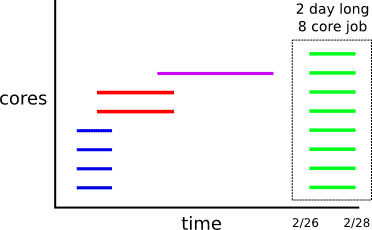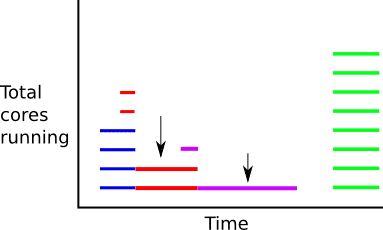# Cost Comparison: Prepaid vs. On DemandRescale offers several price options for running your HPC simulations: On Demand, Low Priority, and Prepaid.  This article will show an analysis of compute usage to determine when getting a prepaid plan makes sense from a cost standpoint.
Our Prepaid price plan provides a long term reservation for Rescale cores at the lowest cost per hour, in exchange for paying for all the hours up-front for the reservation.  Prepaid cores are a great way to lower your hardware costs IF you have high enough utilization. In the following, we will answer 2 questions:

• How high does my utilization need to be for a particular core type in order for Prepaid to save me money?
• Given some schedule of core usage, how many Prepaid cores should I buy to minimize my total hardware costs?

Prepaid cost savings by utilization
The first question is calculated by solving this equation:
Cost savings = Utilization * Total reservation hours * Priceon-demand – Total reservation hours * Priceprepaid
The Prepaid price option offers two time periods to choose from. Users can prepay cores for 1 year or 3 years. So in the case of our popular Nickel core type (which has a 3 year Prepaid cost of \$0.04/core/hour), the break-even point where cost savings = 0 is:
Utilization = \$0.04 per hour Prepaid / \$0.15 per hour On Demand =~ 27%
If your average utilization of a Nickel core is above 27%, Prepaid will save you money compared to On Demand rates.  If your average utilization over a year is 50%, you are saving \$482 per year per core using the Prepaid option over a three year term, instead of On Demand.
Pre-pay how much for a given job forecast?
The above calculation is simple but you may not have a target utilization in mind. Instead, you may have a forecast of all the compute jobs you are planning to run, often based on historical usage. How do we take a schedule of jobs and determine the optimal number of Prepaid cores to buy?
As before, we calculate something similar:
Prepaid savings(x cores Prepaid) = All On Demand cost – (Prepaid cost(x) + Residual On Demand cost(x))
All On Demand cost is the total core hours for all your jobs over the Prepaid period, multiplied by the On Demand core price.
Prepaid cost is just the Prepaid cost for x cores.
Residual On Demand cost is the tricky one to calculate. We need to derive the additional On Demand core hours needed, while maximizing utilization of our Prepaid cores.
To calculate the residual core hours, we need to look at the number of cores running per unit time, and then only consider the cores that are not Prepaid. Visually, we are packing core use time intervals from the bottom up and slicing out the bottom x cores across jobs in time (which are using Prepaid cores) and then just calculating the cost of the rest.The above figure does not take into account that core time intervals will not always either be entirely overlapping or disjoint. The intervals really need to be cut up into smaller disjoint intervals and then we fill from the bottom with these interval parts.Next, we just count up the cores for each time slice, missing slices implicitly have zero cores.Here we have completely covered our reservation period with disjoint intervals. Next, we can subtract off the Prepaid count, for example if we have 2 Prepaid cores, we get these residual core intervals.From these intervals, we then sum the product of the intervals lengths and core counts to get our residual core hours.
Let’s look at how to do this same analysis programmatically. Our inputs are:

• Schedule of compute jobs, which is a list of partially overlapping (start_time, end_time, core_count) tuples
• Start of the prepaid reservation period
• End of the prepaid reservation period
• Number of prepaid cores
```def calculate_residual_core_hours(core_use_intervals,
reservation_start,
reservation_end,
prepaid_core_count):
# sort on start_time
sorted_intervals = sorted(core_use_intervals, key=itemgetter(0))
disjoint_intervals = chop_and_aggregate(reservation_start,
reservation_end,
sorted_intervals)
residual_core_intervals = (
(start_time, end_time, max(0, (count - prepaid_core_count)))
for start_time, end_time, count in disjoint_intervals)
return sum((end_time - start_time) * count
for start_time, end_time, count in residual_core_intervals)
```

Most of the complexity here is hiding in chop_and_aggregate. Let’s look at an implementation of this. We start at reservation_start and step forward in time, keeping track of the intervals that are currently open. When an interval opens or closes, we create a new disjoint interval with the current core count between the last 2 time boundaries..

```def chop_and_aggregate(reservation_start, reservation_end, sorted_intervals):
current_time = reservation_start
open_intervals = Counter()
closed_intervals = []
for start, end, count in sorted_intervals:
# close intervals that end before next interval start
if open_intervals:
next_end = min(open_intervals.keys())
while start >= next_end:
closed_intervals.append((current_time, next_end,
sum(open_intervals.values())))
del open_intervals[next_end]
current_time = next_end
if open_intervals:
next_end = min(open_intervals.keys())
else:
break
# split open intervals at new interval start time
if open_intervals:
closed_intervals.append((current_time, start,
sum(open_intervals.values())))
current_time = start
open_intervals[end] += count
# close out remaining open intervals
for end in sorted(open_intervals.keys()):
end = min(end, reservation_end)
if open_intervals:
closed_intervals.append((current_time, end,
sum(open_intervals.values())))
del open_intervals[end]
return closed_intervals
```

Putting this all together, you can multiply the residual core hours by the On Demand price and get your cost savings.  You can even use calculate_residual_core_hours with zero prepaid cores to (inefficiently) get the all-on-demand cost:

```all_on_demand_hours = calculate_residual_core_hours(job_intervals,
reservation_start,
reservation_end,
0)
residual_on_demand_hours = calculate_residual_core_hours(job_intervals,
reservation_start,
reservation_end,
prepaid_cores)
savings = (all_on_demand_hours * on_demand_price)
- (prepaid_cores * prepaid_price +
residual_on_demand_hours * on_demand_price)
```

From here, you could do a binary search over the number of prepaid_cores to find your optimal savings. As an optimization, the disjoint_intervals only need to be calculated once and for all the different Prepaid core calculations.
•